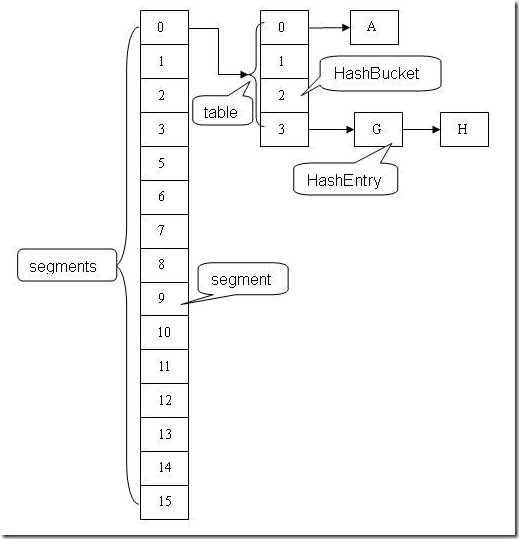# 同步容器和并发容器

### 二、并发容器

#### ConcurrentHashMap

ConcurrentHashMap与HashMap一样是一个哈希表，但是它使用完全不同的锁策略，可以提供更好的并发性和可伸缩性。在ConcurrentHashMap以前，程序使用一个公共锁同步一个方法，并严格地控制只能在一个线程中可以同时访问容器，而ConcurrentHashMap使用一个更为细化的锁机制，名叫分离锁。这个机制允许任意数量的读线程可以并发访问Map，读者和写者也可以并发访问Map，并且有限数量的写进程还可以并发修改Map，结果是为并发访问带来更高的吞吐量，同时几乎没有损失单个线程访问的性能。

ConcurrentHashMap是由Segment数组结构和HashEntry数组结构组成。Segment是一种可重入锁ReentrantLock，在ConcurrentHshMap里扮演锁的角色，HashEntry则用于存储键值对数据。一个ConcurrentHashMap里包含一个Segment数组，Segment的结构和HashMap类似，是一种数组和链表结构，一个Segment里包含一个HashEntry数组，每一个HashEntry是一个链表结构的元素，每个Segment守护着HashEntry数组里的元素，当对HashEntry数组的数据进行修改时，必须首先获得它对应的Segment的锁。HashEntry类用来封装散列表中的键值对。类定义如下：

static final class HashEntry<K,V> {
final int hash;
final K key;
volatile V value;
volatile HashEntry<K,V> next;

HashEntry(int hash, K key, V value, HashEntry<K,V> next) {
this.hash = hash;
this.key = key;
this.value = value;
this.next = next;
}

/**
* Sets next field with volatile write semantics.  (See above
*/
final void setNext(HashEntry<K,V> n) {
UNSAFE.putOrderedObject(this, nextOffset, n);
}

// Unsafe mechanics
static final sun.misc.Unsafe UNSAFE;
static final long nextOffset;
static {
try {
UNSAFE = sun.misc.Unsafe.getUnsafe();
Class k = HashEntry.class;
nextOffset = UNSAFE.objectFieldOffset
(k.getDeclaredField("next"));
} catch (Exception e) {
throw new Error(e);
}
}
}

HashEntry类的value域被声明为Volatile类型，Java的内存模型可以保证：某个写线程对value域的写入可以马上被后续的某个读线程“看”到。

Segment类继承于ReentrantLock，从而使得Segment对象能充当锁的角色。

static final class Segment<K,V> extends ReentrantLock implements Serializable
{
private static final long serialVersionUID = 2249069246763182397L;
static final int MAX_SCAN_RETRIES =
Runtime.getRuntime().availableProcessors() > 1 ? 64 : 1;
transient volatile HashEntry<K,V>[] table;
transient int count; //在本segment范围内，包含的HashEntry的个数
transient int modCount; //table被更新的次数
transient int threshold; //当table中包含的HashEntry元素超过threshold时，触发table再散列
/*
* table是由HashEntry对象组成的数组
* 如果散列时发生碰撞，碰撞的HashEntry对象就以链表的形式链接成一个链表
* table数组的数组成员代表散列映射表的一个桶
* 每一个table守护整个ConcurrentHashMap包含桶总数的一部分
* 如果并发级别为16，table则守护ConcurrentHashMap包含桶总数的1/16
*/
Segment(float lf, int threshold, HashEntry<K,V>[] tab)
{
this.threshold = threshold;
this.table = tab;
}
...
}

ConcurrentHashMap类在默认并发级别会创建包含16个Segment对象的数组 。每个 Segment 的成员对象 table 包含若干个散列表的桶。每个桶是由 HashEntry 链接起来的一个链表。如果键能均匀散列，每个 Segment 大约守护整个散列表中桶总数的 1/16。

//创建一个带有指定初始容量、加载因子和并发级别的空映射
public ConcurrentHashMap(int initialCapacity,float loadFactor, int concurrencyLevel)
{
if (!(loadFactor > 0) || initialCapacity < 0 || concurrencyLevel <= 0)
throw new IllegalArgumentException();
if (concurrencyLevel > MAX_SEGMENTS)
concurrencyLevel = MAX_SEGMENTS;
// Find power-of-two sizes best matching arguments
int sshift = 0;
int ssize = 1;
while (ssize < concurrencyLevel)
{
++sshift;
ssize <<= 1;
}
this.segmentShift = 32 - sshift;  //段偏移量
this.segmentMask = ssize - 1;        //Segment的掩码值，key的散列码的高位来选择具体的Segment
if (initialCapacity > MAXIMUM_CAPACITY)
initialCapacity = MAXIMUM_CAPACITY;
int c = initialCapacity / ssize;
if (c * ssize < initialCapacity)
++c;
int cap = MIN_SEGMENT_TABLE_CAPACITY;
while (cap < c)
cap <<= 1;
//创建 segments和segments
Segment<K,V>[] ss = (Segment<K,V>[])new Segment[ssize];
UNSAFE.putOrderedObject(ss, SBASE, s0); // ordered write of segments
this.segments = ss;
}

public V put(K key, V value)
{
Segment<K,V> s;
if (value == null) //ConcurrentHashMap 中不允许用 null作为映射值，当读到null时，便知道产生了冲突-发生了重排序现象，需加锁后重新读入这个value值
throw new NullPointerException();
int hash = hash(key);
//根据hash值找到相应的Segment
int j = (hash >>> segmentShift) & segmentMask;
if ((s = (Segment<K,V>)UNSAFE.getObject          // nonvolatile; recheck
(segments, (j << SSHIFT) + SBASE)) == null) //  in ensureSegment
s = ensureSegment(j);
return s.put(key, hash, value, false);
}

private Segment<K,V> ensureSegment(int k)
{
final Segment<K,V>[] ss = this.segments;
long u = (k << SSHIFT) + SBASE; // raw offset
Segment<K,V> seg;
if ((seg = (Segment<K,V>)UNSAFE.getObjectVolatile(ss, u)) == null)
{
Segment<K,V> proto = ss; // use segment 0 as prototype
int cap = proto.table.length;
int threshold = (int)(cap * lf);
HashEntry<K,V>[] tab = (HashEntry<K,V>[])new HashEntry[cap];
if ((seg = (Segment<K,V>)UNSAFE.getObjectVolatile(ss, u))== null)
{ // recheck
Segment<K,V> s = new Segment<K,V>(lf, threshold, tab);
while ((seg = (Segment<K,V>)UNSAFE.getObjectVolatile(ss, u))== null)
{
if (UNSAFE.compareAndSwapObject(ss, u, null, seg = s))
break;
}
}
}
return seg;
}

final V put(K key, int hash, V value, boolean onlyIfAbsent)
{
HashEntry<K,V> node = tryLock() ? null :scanAndLockForPut(key, hash, value);//加锁
V oldValue;
try
{
HashEntry<K,V>[] tab = table;
/*
* 把hash值与table数组的长度减1的值相与
* 得到该hash值对应的table数组的下标值
*/
int index = (tab.length - 1) & hash;
//找到hash值对应的具体的那个桶
HashEntry<K,V> first = entryAt(tab, index);
for (HashEntry<K,V> e = first;;)
{
if (e != null)  //如果键值对已经存在
{
K k;
if ((k = e.key) == key ||(e.hash == hash && key.equals(k)))
{
oldValue = e.value;
if (!onlyIfAbsent)
{
e.value = value;
++modCount;
}
break;
}
e = e.next;
}
else //键值对不存在
{
if (node != null)
node.setNext(first);
else
node = new HashEntry<K,V>(hash, key, value, first);//创建新的节点到链表中
int c = count + 1;
if (c > threshold && tab.length < MAXIMUM_CAPACITY)
rehash(node);
else
setEntryAt(tab, index, node);
++modCount;
count = c;
oldValue = null;
break;
}
}
}
finally
{
unlock(); //解锁
}
return oldValue;
}

public V remove(Object key)
{
int hash = hash(key);
Segment<K,V> s = segmentForHash(hash);
return s == null ? null : s.remove(key, hash, null);
}

final V remove(Object key, int hash, Object value)
{
if (!tryLock())    //加锁
scanAndLock(key, hash);
V oldValue = null;
try {
HashEntry<K,V>[] tab = table;
//根据hash值找到table的下标值
int index = (tab.length - 1) & hash;
//找到hash对应的那个桶
HashEntry<K,V> e = entryAt(tab, index);
HashEntry<K,V> pred = null;
while (e != null)
{
K k;
HashEntry<K,V> next = e.next;
if ((k = e.key) == key ||(e.hash == hash && key.equals(k)))
{
V v = e.value;
if (value == null || value == v || value.equals(v)) //找到要删除的节点
{
if (pred == null)
setEntryAt(tab, index, next);
else
pred.setNext(next);
++modCount;
--count;
oldValue = v;
}
break;
}
pred = e;
e = next;
}
} finally {
unlock(); //解锁
}
return oldValue;
}

clear操作的源码如下，对每个段Segment进行遍历，然后进行clear操作:

public void clear()
{
final Segment<K,V>[] segments = this.segments;
for (int j = 0; j < segments.length; ++j)
{
Segment<K,V> s = segmentAt(segments, j);
if (s != null)
s.clear();
}
}
final void clear()
{
lock();
try
{
HashEntry<K,V>[] tab = table;
for (int i = 0; i < tab.length ; i++)
setEntryAt(tab, i, null);
++modCount;
count = 0;
}
finally
{
unlock();
}
}

### 三、总结

ConcurrentHashMap 是一个并发散列映射表的实现，它允许完全并发的读取，并且支持给定数量的并发更新。相比于 HashTable 和用同步包装器包装的 HashMap（Collections.synchronizedMap(new HashMap())），ConcurrentHashMap 拥有更高的并发性。在 HashTable 和由同步包装器包装的 HashMap 中，使用一个全局的锁来同步不同线程间的并发访问。同一时间点，只能有一个线程持有锁，也就是说在同一时间点，只能有一个线程能访问容器。

ConcurrentHashMap 的高并发性主要来自于三个方面：

• 用分离锁实现多个线程间的更深层次的共享访问。
• 用 HashEntery 对象的不变性来降低执行读操作的线程在遍历链表期间对加锁的需求。
• 通过对同一个 Volatile 变量的写 / 读访问，协调不同线程间读 / 写操作的内存可见性。

######

posted @ 2019-03-04 23:12  robin·张  阅读(...)  评论(...编辑  收藏